# Paired Two-sample T-test (Dependent T-test)

What is a Paired 2-sample T-test?

Let’s analyze this definition from scratch.

• A T-test is a statistical test whose outcomes follow a T-distribution.
• Two-sample means we have 2 sets of samples, and our target is to verify if the means of the 2 distributions that generate these 2 sample sets are equal.
• Paired means these 2 sample sets are not independent of each other, each observation in one sample set must correspond to one and only one observation in the other set.

Thus, in summary, a Paired 2-sample T-test takes as input 2 sample sets that have their observations linked to the other on a 1-to-1 basis, and the test’s outputs follow a T-distribution. This is also abbreviated as the Paired T-test or Dependent T-test.

In contrast to the Paired 2-sample T-test, we also have the Unpaired 2-sample T-test.

Notice that the result taken from the Paired Test is more significant than from the Unpaired Test given the same samples (because the 1-to-1 relationship gives additional information), it is recommended to take the Paired Test if possible (i.e. if the conditions for a Paired Test hold).

Assumptions

The Paired 2-sample T-test is a parametric test, thus it requires some assumptions to be true (or at least approximately true):

• The observations must be measured in numerical values (i.e. continuous, interval or ratio). For categorical variables, we should use another test, for example, the Chi-squared test.
• The distribution that generates the differences between paired values must be a Normal distribution. This normality can be roughly verified by examining the differences using, for instance, QQ-plot, Shapiro-Wilk or Anderson Darling test. For non-normal data, we can either try to transform it to normal or use a non-parametric test, e.g. Mann Whitney Test and Wilcoxon Signed Rank Test. Note that we do NOT need each set to follow Normal distribution, but rather the differences must (approximately) normally distributed.
• Each observation must be independent of the others in the same sample set. Dependencies between observations in the same set may affect the objectivity of the test, which makes the test result unreliable. This assumption is almost always attached to all, including both parametric and non-parametric tests.
• There must not be any big-influencers (outliers). Outliers may bias the test, especially when the sample size is small (, which case is often for medical tests). In cases there are big-influencers in our data, we may choose to remove it (with care) or switch to a robust test like the Wilcoxon Signed Rank Test, which calculates the ranking thus is not impacted so much by extreme values.

Note that even though the tests’ general goal is to check if the means of the 2 distributions are equal or not, it is usually the case that it checks whether the 2 distributions are the same or not. That is, we usually assume the variances of the 2 distribution are equal, then we test if the means are also equal (both the means and variances are equal indicates that the 2 distributions are also the same) or not (implies that 2 distributions are not the same).

Conduct the Test

The Paired 2-sample T-test is just a One-sample T-test in disguise. Put it another way, we can transform the Paired T-test into a One-sample T-test.

This transformation can be elaborated by restating the problem: we want to test if the 2 sample sets are generated by the same distribution, which is identical to test if the differences between them are generated by a distribution with mean 0.

Call:

• n as the size of each sample set.
•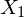and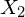as the 2 sets, where the i-th observation of(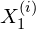) is related to the i-th observation of(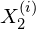),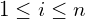.
•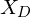as the set of differences between each observation inwith the correspondence in. (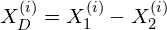.)
•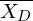is the mean of.
•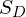is the sample standard deviation of. Note that this is the sample std, so we compute the unbiased std (i.e. divide by n-1 instead of n).

Here, the T-statistic is taken by: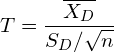with the degree of freedom DF = n – 1.

After getting the T-statistics and the degree of freedom, we can verify our hypothesis using the T-table (or with the help of Python, or other means) as previously described here.

Example

Suppose we want to measure the effectiveness of a diet. We gauss the weights of 10 people before and after practicing the diet to verify if there is any statistical difference. The weights are shown in the table below, where each row represents 1 person.

Let’s suppose our significance level (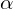) is 0.05.

To solve this problem, firstly, we make the set of differences,.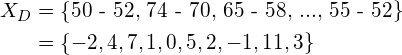Secondly, we calculate the mean and standard deviation of this set: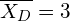The T-statistic is then: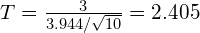with the degree of freedom DF = n – 1 = 9.

Look up the T-table, we take it that the Critical Value forof a 2-tailed test with DF = 9 is 2.262, which is smaller than our T-statistic 2.405. Hence, we conclude that the impact of the diet is statistically significant.

References: